# Please answer me in detail. Thank you. Question 3 Consider a production function of three inputs, labor, capital, and m...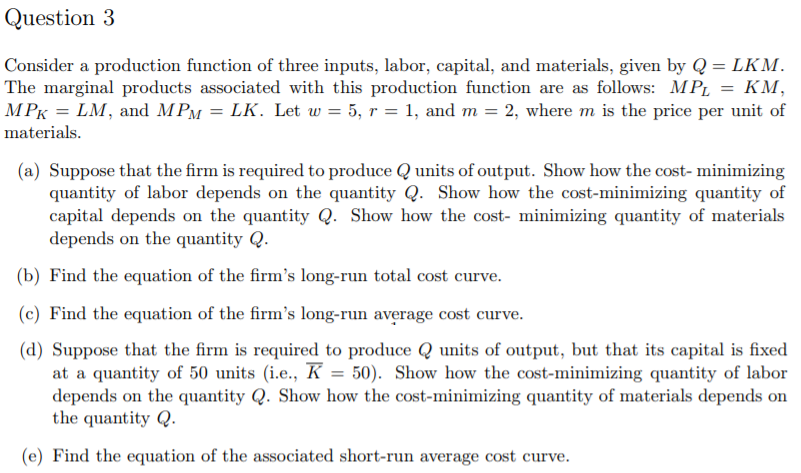Question 3 Consider a production function of three inputs, labor, capital, and materials, given by Q-LKM The marginal products associated with this production function are as follows: MPLKM MPK-LM, and MPM = LK. Let w 5, r = 1, and m = 2, where m is the price per unit of materials. (a) Suppose that the firm is required to produce Qunits of output. Show how the cost-minimizing quantity of labor depends on the quantity Q. Show how the cost-minimizing quantity of capital depends on the quantity Q. Show how the cost- minimizing quantity of materials depends on the quantity Q. (b) Find the equation of the firm's long-run total cost curve (c) Find the equation of the firm's long-run average cost curve. (d) Suppose that the firm is required to produce Q units of output, but that its capital is fixed at a quantity of 50 units (i.e., K50). Show how the cost-minimizing quantity of labor depends on the quantity Q. Show how the cost-minimizing quantity of materials depends on the quantityQ e) Find the equation of the associated short-run average cost curve.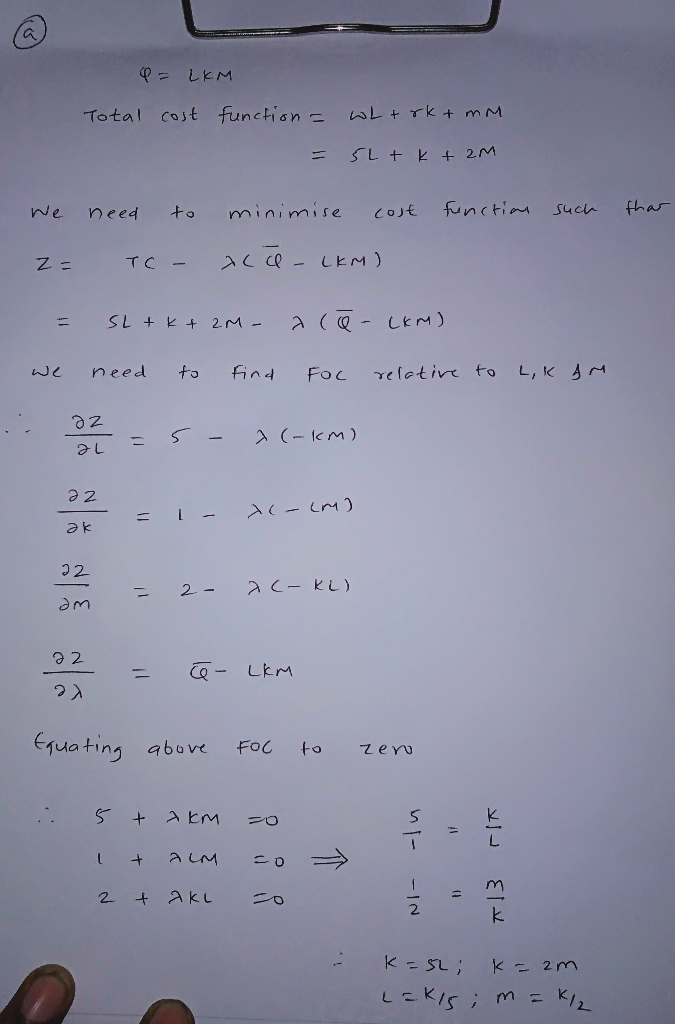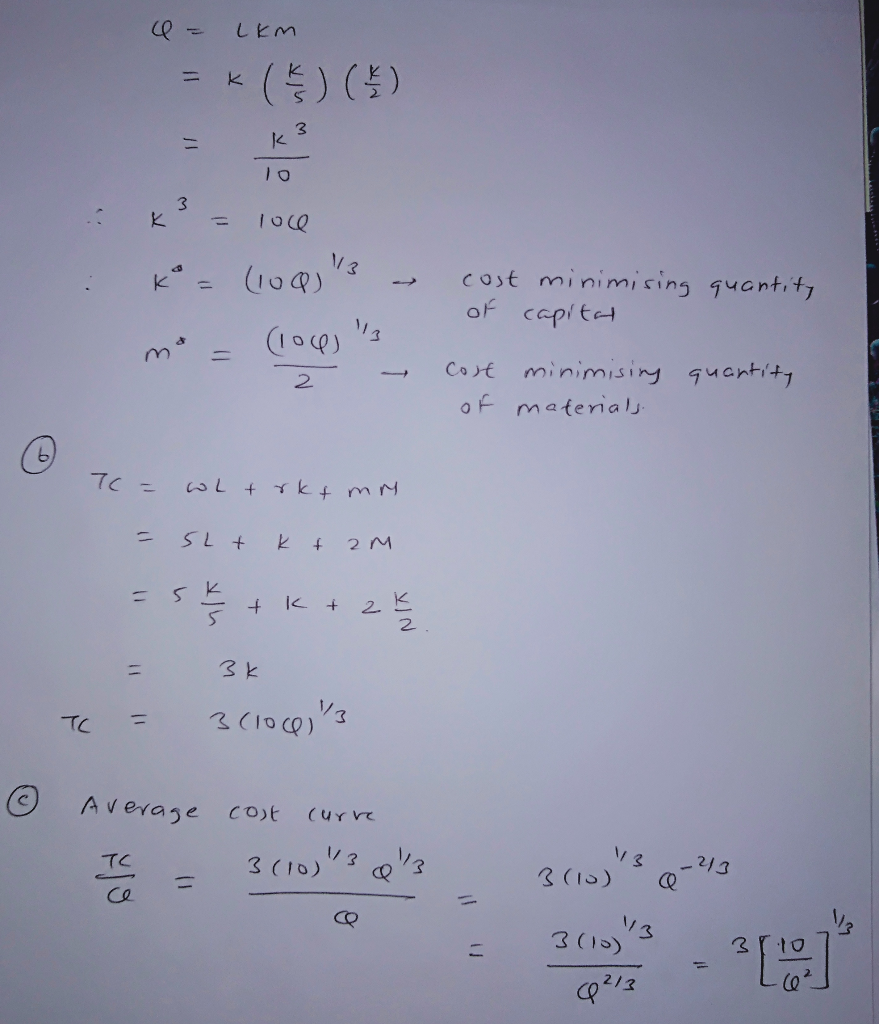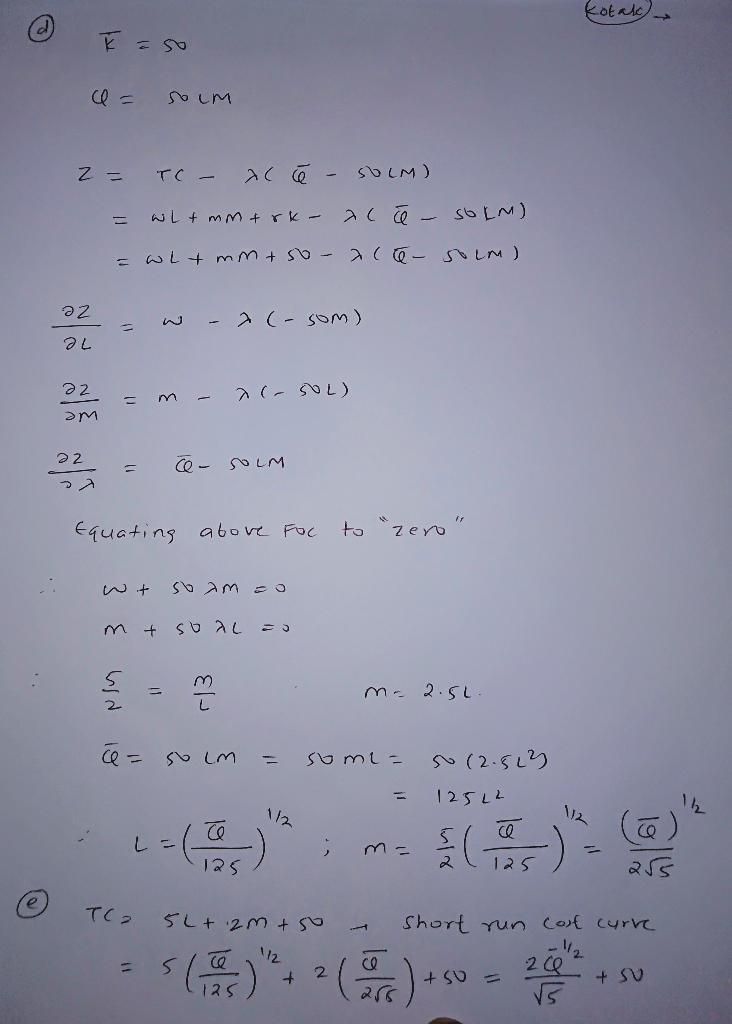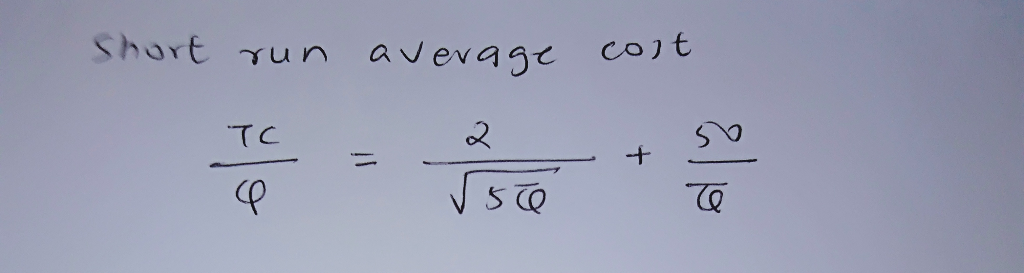##### Add Answer of: Please answer me in detail. Thank you. Question 3 Consider a production function of three inputs, labor, capital, and m...
More Homework Help Questions Additional questions in this topic.